Students can download Maths Chapter 5 Coordinate Geometry Ex 5.3 Questions and Answers, Notes, Samacheer Kalvi 10th Maths Guide Pdf helps you to revise the complete Tamilnadu State Board New Syllabus, helps students complete homework assignments and to score high marks in board exams.

## Tamilnadu Samacheer Kalvi 10th Maths Solutions Chapter 5 Coordinate Geometry Ex 5.3

Question 1.
Find the equation of a straight line passing through the mid-point of a line segment joining the points (1, -5), (4, 2) and parallel to (i) X axis (ii) Y axis
Solution:
Mid point of the line joining to points (1, -5), (4, 2)
Mid point of the line = ($$\frac{x_{1}+x_{2}}{2}, \frac{y_{1}+y_{2}}{2}$$)
= ($$\frac { 1+4 }{ 2 }$$,$$\frac { -5+2 }{ 2 }$$) = ($$\frac { 5 }{ 2 }$$,$$\frac { -3 }{ 2 }$$)

(i) Any line parallel to X-axis. Slope of a line is 0.
Equation of a line is y – y1 = m (x – x1)
y + $$\frac { 3 }{ 2 }$$ = 0 (x – $$\frac { 5 }{ 2 }$$)
y + $$\frac { 3 }{ 2 }$$ = 0 ⇒ $$\frac { 2y+3 }{ 2 }$$ = 0
2y + 3 = 0

(ii) Equation of a line parallel to Y-axis is
x = $$\frac { 5 }{ 2 }$$ ⇒ 2x = 5
2x – 5 = 0Question 2.
The equation of a straight line is 2(x – y) + 5 = 0. Find its slope, inclination and intercept on the Y axis.
Solution:
Equation of a line is
2 (x – y) + 5 = 0
2 x – 2y + 5 = 0
-2y = -2x – 5
2y = 2x + 5
y = $$\frac { 2x }{ 2 }$$ + $$\frac { 5 }{ 2 }$$
y = x + $$\frac { 5 }{ 2 }$$
Slope of line = 1
Y intercept = $$\frac { 5 }{ 2 }$$
tan θ = 1
tan θ = tan 45°
∴ angle of inclination = 45°Question 3.
Find the equation of a line whose inclination is 30° and making an intercept -3 on the Y axis.
Solution:
Angle of inclination = 30°
Slope of a line = tan 30°
(m) = $$\frac{1}{\sqrt{3}}$$
y intercept (c) = -3
Equation of a line is y = mx + c
y = $$\frac{1}{\sqrt{3}}$$ x – 3
$$\sqrt { 3 }$$ y = x – 3 $$\sqrt { 3 }$$
∴ x – $$\sqrt { 3 }$$ y – 3 $$\sqrt { 3 }$$ = 0

Question 4.
Find the slope and y intercept of $$\sqrt { 3x }$$ + (1 – $$\sqrt { 3 }$$)y = 3.
Solution:
The equation of a line is $$\sqrt { 3 }$$x + (1 – $$\sqrt { 3 }$$)y = 3
(1 – $$\sqrt { 3 }$$)y = $$\sqrt { 3 }$$ x + 3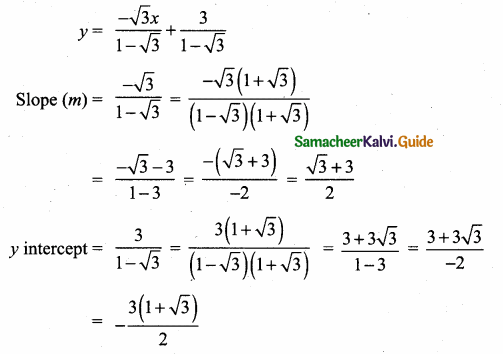Question 5.
Find the value of ‘a’, if the line through (-2,3) and (8,5) is perpendicular to y = ax + 2
Solution:
Given points are (-2, 3) and (8, 5)
Slope of a line = $$\frac{y_{2}-y_{1}}{x_{2}-x_{1}}$$
= $$\frac { 5-3 }{ 8+2 }$$ = $$\frac { 2 }{ 10 }$$ = $$\frac { 1 }{ 5 }$$
Slope of a line y = ax + 2 is “a”
Since two lines are ⊥r
m1 × m2 = -1
$$\frac { 1 }{ 5 }$$ × a = -1 ⇒ $$\frac { a }{ 5 }$$ = -1 ⇒ a = -5
∴ The value of a = -5

Question 6.
The hill in the form of a right triangle has its foot at (19,3). The inclination of the hill to the ground is 45°. Find the equation of the hill joining the foot and top.
Solution:
Slope of AB (m) = tan 45°
= 1
Equation of the hill joining the foot and the top is
y – y1 = m(x – x1)
y – 3 = 1 (x – 19)y – 3 = x – 19
– x + y – 3 + 19 = 0
– x + y + 16 = 0
x – y – 16 = 0
The required equation is x – y – 16 = 0Question 7.
Find the equation of a line through the given pair of points.
(i) (2,$$\frac { 2 }{ 3 }$$) and ($$\frac { -1 }{ 2 }$$,-2)
(ii) (2,3) and (-7,-1)
Solution:
(i) Equation of the line passing through the point (2,$$\frac { 2 }{ 3 }$$) and ($$\frac { -1 }{ 2 }$$,-2)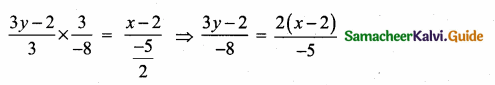-5(3y – 2) = -8 × 2 (x – 2)
-15y + 10= -16 (x – 2)
-15y + 10= -16x + 32
16x – 15y + 10 – 32 = 0
16x – 15y – 22 = 0
The required equation is 16x – 15y – 22 = 0

(ii) Equation of the line joining the point (2, 3) and (-7, -1) is-9 (y – 3) = -4 (x – 2)
-9y + 27 = – 4x + 8
4x – 9y + 27 – 8 = 0
4x – 9y + 19 = 0
The required equation is 4x – 9y + 19 = 0Question 8.
A cat is located at the point(-6, -4) in xy plane. A bottle of milk is kept at (5,11). The cat wish to consume the milk travelling through shortest possible distance. Find the equation of the path it needs to take its milk.
Solution: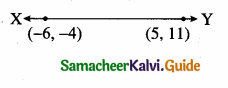Equation of the line joining the point is
$$\frac{y-y_{1}}{y_{2}-y_{1}}=\frac{x-x_{1}}{x_{2}-x_{1}}$$
$$\frac { y+4 }{ 11+4 }$$ = $$\frac { x+6 }{ 5+6 }$$
$$\frac { y+4 }{ 15 }$$ = $$\frac { x+6 }{ 11 }$$
15(x + 6) = 11(y + 4)
15x + 90 = 11y + 44
15x – 11y + 90 – 44 = 0
15x – 11y + 46 = 0
The equation of the path is 15x – 11y + 46 = 0

Question 9.
Find the equation of the median and altitude of AABC through A where the vertices are A(6,2), B(-5, -1) and C(1,9).
Solution:
(i) To find medianEquation of the median AD is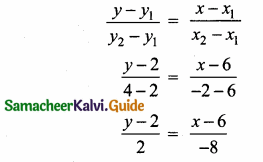2(x – 6) = -8 (y – 2)
2x – 12= -8y + 16
2x + 8y – 28 = 0
(÷ by 2) x + 4y – 14 = 0
∴ Equation of the median is x + 4y – 14 = 0
Equation of the altitude is 3x + 5y – 28 = 0

(ii) To find the equation of the altitude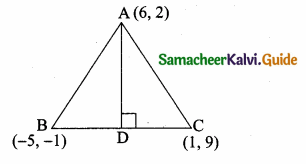Slope of BC = $$\frac{y_{2}-y_{1}}{x_{2}-x_{1}}$$
= $$\frac { 9+1 }{ 1+5 }$$
= $$\frac { 10 }{ 6 }$$ = $$\frac { 5 }{ 3 }$$
Slope of the altitude = – $$\frac { 3 }{ 5 }$$
Equation of the altitude AD is
y – y1 = m (x – x1)
y – 2 = – $$\frac { 3 }{ 5 }$$ (x – 6)
-3 (x – 6) = 5 (y – 2)
-3x + 18 = 5y – 10
-3x – 5y + 18 + 10 = 0
-3x – 5y + 28 = 0
3x + 5y – 28 = 0Question 10.
Find the equation of a straight line which has slope $$\frac { -5 }{ 4 }$$ and passing through the point (-1,2).
Solution:
Slope of a line (m) = $$\frac { -5 }{ 4 }$$
The given point (x1, y1) = (-1, 2)
Equation of a line is y – y1 = m (x – x1)
y – 2= $$\frac { -5 }{ 4 }$$ (x + 1)
5(x + 1) = -4(y – 2)
5x +5 = -4y + 8
5x + 4y + 5 – 8 = 0
5x + 4y – 3 = 0
The equation of a line is 5x + 4y – 3 = 0Question 11.
You are downloading a song. The percenty (in decimal form) of mega bytes remaining to get downloaded in x seconds is given by y = -0.1x + 1.
(i) graph the equation.
(ii) find the total MB of the song.
(iii) after how many seconds will 75% of the song gets downloaded?
Solution:
(i) y = – 0. 1x + 1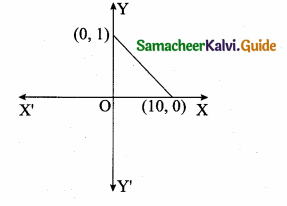(ii) y = -0.1x + 1
x → seconds
y → Mega byte of the song.
The total mega byte of the song is at the beginning that is when x = 0
y = 1 mega byte

In other words y = 25%
y = 0.25
By using the equation we get
0.25 = -0.1x + 1
0.1 x = 0.75
x = $$\frac { 0.75 }{ 0.1 }$$ = 7.5 seconds

i.e y = 0
0 = -0.1 x + 1
0.1 x = 1 second
x = $$\frac { 1 }{ 0.1 }$$ = $$\frac { 1 }{ 1 }$$ × 10
x = 10 secondsQuestion 12.
Find the equation of a line whose intercepts on the x and y axes are given below.
(i) 4, -6
(ii) -5, – 4
Solution:
(i) x intercept (a) = 4; y intercept (b) = – 6
Equation of a line is $$\frac { x }{ a }$$ + $$\frac { y }{ b }$$ = 1
$$\frac { x }{ 4 }$$ + $$\frac { y }{ -6 }$$ = 1 ⇒ $$\frac { x }{ 4 }$$ – $$\frac { y }{ 6 }$$ = 1
(LCM of 4 and 6 is 12)
3x – 2y = 12
3x – 2y – 12 = 0
The equation of a line is 3x – 2y – 12 = 0

(ii) x intercept (a) = -5; y intercept (b) = $$\frac { 3 }{ 4 }$$
Equation of a line is $$\frac { x }{ a }$$ + $$\frac { y }{ b }$$ = 1 ⇒ $$\frac{x}{-5}+\frac{y}{\frac{3}{4}}=1$$
$$\frac { x }{ -5 }$$ + $$\frac { 4y }{ 3 }$$ = 1
(LCM of 5 and 3 is 15)
– 3x + 20y = 15
– 3x + 20y – 15 = 0
3x – 20y + 15 = 0
∴ Equation of a line is 3x – 20y + 15 = 0Question 13.
Find the intercepts made by the following lines on the coordinate axes.
(i) 3x – 2y – 6 = 0
(ii) 4x + 3y + 12 = 0
Solution:
(i) 3x – 2y – 6 =0.
x intercept when y = 0
⇒ 3x – 6 = 0
⇒ x = 2
y intercept when x = 0
⇒ 0 – 2y – 6 = 0
⇒ y = -3
(ii) 4x + 3y + 12 = 0. x intercept when y = 0
⇒ 4x + 0 + 12 = 0
⇒ x = -3
y intercept when x = 0
⇒ 0 + 3y + 12 = 0
⇒ y = -4Question 14.
Find the equation of a straight line.
(i) passing through (1,-4) and has intercepts which are in the ratio 2 : 5
(ii) passing through (-8, 4) and making equal intercepts on the coordinate axes.
Solution:
(i) Let the x-intercept be 2a and the y intercept 5 a
The equation of a line is
$$\frac { x }{ a }$$ + $$\frac { y }{ a }$$ = 1 ⇒ $$\frac { x }{ 2a }$$ + $$\frac { y }{ 5a }$$ = 1
The line passes through the point (1, -4)
$$\frac { 1 }{ 2a }$$ + $$\frac { (-4) }{ 5a }$$ = 1 ⇒ $$\frac { 1 }{ 2a }$$ – $$\frac { 4 }{ 5a }$$ = 1
Multiply by 10a
(L.C.M of 2a and 5a is 10a)
5 – 8 = 10a ⇒ -3 = 10a
a = $$\frac { -3 }{ 10 }$$
The equation of the line is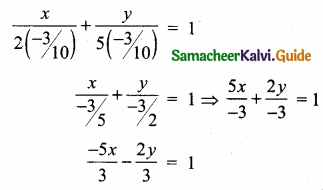Multiply by 3
-5x – 2y = 3 ⇒ -5x – 2y – 3 = 0
5x + 2y + 3 = 0
The equation of a line is 5x + 2y + 3 = 0

(ii) Let the x-intercept andy intercept “a”
The equation of a line is
$$\frac { x }{ a }$$ + $$\frac { y }{ a }$$ = 1
The line passes through the point (-8, 4)
$$\frac { -8 }{ a }$$ + $$\frac { 4 }{ a }$$ = 1
$$\frac { -8+4 }{ a }$$ = 1
-4 = a
The equation of a line is
$$\frac { x }{ -4 }$$ + $$\frac { y }{ -4 }$$ = 1
Multiply by -4
x + y = -4
x + y + 4 = 0
The equation of the line is x + y + 4 = 0